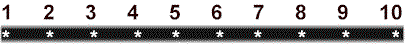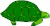Grade One Math Name Number Sense and Numeration Date
 Computation: Subtraction Sentences Title: Subtraction Turtles Skill: Represent subtraction sentences using a counter.

Use This Counter to Help Solve Your EquationsSubtract the number 5 from each of the following numbers. Write your answer below. Subtract the number 3 from each of the following numbers. Write your answer below. 6 - 5 = 1 4 - 3 = 1 8 7 9 6 5 3 7 9 10 10

Turtle Equation: Write the subtraction sentence for the following turtle equation.-=- =

 This lesson may be photocopied for non-commercial educational uses only. Copyright Rodriguez and Simpson, 1999.